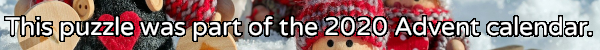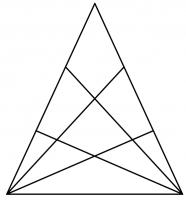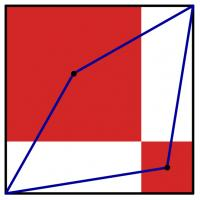mscroggs.co.uk
mscroggs.co.uksubscribe

## 25 DecemberIt's nearly Christmas and something terrible has happened: you've just landed in a town in the Arctic circle with a massive bag of letters for Santa, but you've lost to instructions for how to get to Santa's house near the north pole. You need to work out where he lives and deliver the letters to him before Christmas is ruined for everyone.
Due to magnetic compasses being hard to use near the north pole, you brought with you a special Advent compass. This compass has nine numbered directions. Santa has given the residents of the town clues about a sequence of directions that will lead to his house; but in order to keep his location secret from present thieves, he gave each resident two clues: one clue is true, and one clue is false.
Here are the clues:
9
"The 4th digit is 4."
"The 8th digit is 4."
4
"The 3rd digit is 1."
"The 4th digit is 7."
10
"The 11th digit is a factor of 888."
"The 11th digit is a factor of 88."
13
"The 3rd digit is 1."
"The 4th digit is 6."
23
"The 10th digit is 4."
"The 10th digit is 3."
1
"The last digit is 1, 9 or 5."
"The last digit is 1."
5
"The 3rd digit is 1."
"The 4th digit is 3."
16
"The 5th digit is 3."
"The 6th digit is 3."
11
"The sequence's length is 2+1+6."
"The sequence's length is 2×1×6."
12
"The 6th to 8th digits are 3, 3, 4."
"The 7th to 9th digits are 3, 3, 4."
2
"The 7th digit is 4."
"The 8th digit is 4."
21
"The 5th digit is 3."
"The 9th digit is 9."
22
"The 9th digit is 9."
"The 10th digit is 4."
17
"The 3rd digit is 1."
"The 4th digit is 9."
15
"The 2nd* digit is 7."
"The 1st digit is 8."
14
"The 1st digit is 7."
"The 2nd digit is 7."
7
"The last digit is not 5."
"The 4th digit is 5."
3
"The 11th digit is a factor of 10."
"The 11th digit is a factor of 321."
6
"The sequence's length is 1+9+2."
"The sequence's length is 1×9×2."
20
"The 5th digit is 3."
"The 5th digit is 4."
19
"5 does not appear."
"5 appears exactly once."
24
"The 3rd digit is 1."
"The 4th digit is 8."
25
18
"The 1st digit is 3, 7 or 8."
"The sequence's length is prime."
8
"The 3rd digit is 1."
"The 4th digit is 2."
You can view the map here.

## 24 DecemberThere are six ways to put two tokens in a 3 by 3 grid so that the diagonal from the top left to the bottom right is a line of symmetry:
Today's number is the number of ways of placing two tokens in a 29 by 29 grid so that the diagonal from the top left to the bottom right is a line of symmetry.

## 23 December198 is the smallest number that is equal to 11 times the sum of its digits.
Today's number is the smallest number that is equal to 48 times the sum of its digits.

## 22 DecemberPut the digits 1 to 9 (using each digit exactly once) in the boxes so that the sums are correct. The sums should be read left to right and top to bottom ignoring the usual order of operations. For example, 4+3×2 is 14, not 10. Today's number is the largest number you can make with the digits in the red boxes.
 + + = 18 + + + ÷ - = 1/2 + + + + ÷ = 3/2 =24 =8 =13
Tags: numbers, grids

## 21 DecemberThere are 3 ways to order the numbers 1 to 3 so that no number immediately follows the number one less that itself:
• 3, 2, 1
• 1, 3, 2
• 2, 1, 3
Today's number is the number of ways to order the numbers 1 to 6 so that no number immediately follows the number one less that itself.

## 20 December18 can be written as the sum of 3 consecutive (strictly) positive integers: 5 + 6 + 7.
18 can also be written as the sum of 4 consecutive (strictly) positive integers: 3 + 4 + 5 + 6.
18 is in fact the smallest number that can be written as the sum of both 3 and 4 consecutive (strictly) positive integers.
Today's number is the smallest number that can be written as the sum of both 12 and 13 consecutive (strictly) positive integers.
Tags: numbers, sums

## 19 DecemberThe diagram to the right shows a triangle. Two of the sides of the triangle have been split into three pieces, with lines drawn from the opposite vertex. In total, the diagram now contains 27 triangles of any size.
Another triangle has two of its sides split into eight pieces, with lines drawn from the opposite vertex. How many triangles (of any size) would this create?

## 18 DecemberThe expansion of $$(x+y+z)^3$$ is
$$x^3 + y^3 + z^3 + 3x^2y + 3x^2z + 3xy^2 + 3y^2z + 3xz^2 + 3yz^2 + 6xyz.$$
This has 10 terms.
Today's number is the number of terms in the expansion of $$(x+y+z)^{26}$$.
Tags: algebra

## 17 DecemberPut the digits 1 to 9 (using each digit exactly once) in the boxes so that the sums and product are correct. Today's number is the product of the numbers in the red boxes.
 + + = 16 + + + + + = 8 + + + × × = 288 =11 =14 =20
Tags: numbers, grids

## 16 DecemberSolve the crossnumber to find today's number. No number starts with 0.

## 15 DecemberWhen talking to someone about this Advent calendar, you told them that the combination of XMAS and MATHS is GREAT. They were American, so asked you if the combination of XMAS and MATH is great; you said SURE. You asked them their name; they said SAM.
Each of the letters E, X, M, A, T, H, S, R, U, and G stands for a different digit 0 to 9. The following sums are correct:
Today's number is SAM. To help you get started, the letter T represents 4.

## 14 DecemberThe numbers 33, 404 and 311 contain duplicate digits. The numbers 120, 15 and 312 do not.
How many numbers between 10 and 999 (inclusive) contain no duplicate digits?

## 13 DecemberThere are 6 ways to split the sequence of the numbers 1 to 5 into three shorter sequences:
• 1 and 2 and 3, 4, 5
• 1 and 2, 3 and 4, 5
• 1 and 2, 3, 4 and 5
• 1, 2 and 3 and 4, 5
• 1, 2 and 3, 4 and 5
• 1, 2, 3 and 4 and 5
Today's number is the number of ways to split the sequence of the numbers 1 to 10 into five shorter sequences.

## 12 DecemberThe diagram to the left shows a large black square. Inside this square, two red squares have been drawn. (The sides of the red squares are parallel to the sides of the black square; each red square shares a vertex with the black square; and the two red squares share a vertex.) A blue quadrilateral has then been drawn with vertices at two corners of the black square and the centres of the red squares.
The area of the blue quadrilateral is 167. What is the area of the black square?

## 11 DecemberNoel has a large pile of cards. Half of them are red, the other half are black. Noel splits the cards into two piles: pile A and pile B.
Two thirds of the cards in pile A are red. Noel then moves 108 red cards from pile A to pile B. After this move, two thirds of the cards in pile B are red.
Note: There was a mistake in the original version of today's puzzle. The number 21 has been replaced with 108.

## 10 DecemberToday's number is the smallest multiple of 24 whose digits add up to 24.

## 9 DecemberPut the digits 1 to 9 (using each digit exactly once) in the boxes so that the sums are correct. The sums should be read left to right and top to bottom ignoring the usual order of operations. For example, 4+3×2 is 14, not 10. Today's number is the product of the numbers in the red boxes.
 + × = 54 × + ÷ - ÷ = 1 ÷ - × + - = 6 =18 =6 =18
Tags: numbers, grids

## 8 DecemberThe residents of Octingham have 8 fingers. Instead of counting in base ten, they count in base eight: the digits of their numbers represent ones, eights, sixty-fours, two-hundred-and-fifty-sixes, etc instead of ones, tens, hundreds, thousands, etc.
For example, a residents of Octingham would say 12, 22 and 52 instead of our usual numbers 10, 18 and 42.
Today's number is what a resident of Octingham would call 11 squared (where the 11 is also written using the Octingham number system).

## 7 DecemberThere are 15 dominos that can be made using the numbers 0 to 4 (inclusive):
The sum of all the numbers on all these dominos is 60.
Today's number is the sum of all the numbers on all the dominos that can be made using the numbers 5 to 10 (inclusive).

## 6 DecemberThere are 12 ways of placing 2 tokens on a 2×4 grid so that no two tokens are next to each other horizonally, vertically or diagonally:
Today's number is the number of ways of placing 5 tokens on a 2×10 grid so that no two tokens are next to each other horizonally, vertically or diagonally.

## 5 DecemberCarol rolled a large handful of six-sided dice. The total of all the numbers Carol got was 521. After some calculating, Carol worked out that the probability that of her total being 521 was the same as the probability that her total being 200. How many dice did Carol roll?

## 4 DecemberToday's number is a three digit number which is equal to the sum of the cubes of its digits. One less than today's number also has this property.

## 3 DecemberPut the digits 1 to 9 (using each digit exactly once) in the boxes so that the sums are correct. The sums should be read left to right and top to bottom ignoring the usual order of operations. For example, 4+3×2 is 14, not 10. Today's number is the largest number you can make with the digits in the red boxes.
 ÷ - = 3 + + ÷ ÷ × = 1 × - + - × = 20 =91 =6 =8
Tags: numbers, grids

## 2 DecemberCarol draws a square with area 62. She then draws the smallest possible circle that this square is contained inside. Next, she draws the smallest possible square that her circle is contained inside. What is the area of her second square?

## 1 DecemberIt is possible to write 325 different numbers using the digits 1, 2, 3, 4, and 5 at most once each (and using no other digits). How many of these numbers are odd?

## Archive

Show me a random puzzle
▼ show ▼